Logarithmic functions are often used when dealing with exponential values in your data. So when you have a log function, you might probably need to find the antilog at some point too.

Unfortunately, Excel does not have a dedicated function to find the antilog of a value.

But there is, of course, an easy way around it. And this is quite intuitive if you understand how logs and antilogs are basically related to one another.

In this tutorial, I will give a brief overview of the basic math behind logs and antilogs, and then I will show you how to use this to quickly find the antilog of any number in Excel.

## What does the Log of a Number Tell You?

In simple words, the log of a number is the number of times a factor occurs in the number in repeated multiplication. The factor that is getting repeatedly multiplied is known as the base of the logarithm.

So, if you want to know what is the log of the number 1000 to the base 10, you are actually finding out how many times the number 10 needs to be multiplied to get 1000.

Since you need to multiply it 3 times to get 1000, i.e:

`10 x 10 x 10 = 1000`

That means the log to the base 10 of 1000 is 3! This can also be symbolically expressed as:

`Log10(1000) = 3`

Logarithms, therefore, are usually used to express really large numbers in fewer digits.

For example, in the above case, we expressed the number 1000 in just a single-digit number, 3.

Similarly, we could express 100,000 with the number 6, which is a single digit too! Imagine how easy it then gets to express really large numbers.

In Excel, we use the LOG10 function to find the log of a number to the base 10.

So if you type: =LOG10(1000) in a given cell, you should get the result as 3.

And if you want to specify the base yourself as well, you can use the LOG function. For example, =LOG(81,3) would give you 3 (where 3×4 is 81)

## What is the Relationship between Log and Antilog?

The antilog of a number, in Excel, is the inverse of the log of a number (to the base 10).

In other words, it is the inverse of the LOG10 function (or Log function if using a base other than 10).

So, to find the antilog of a number, all you need to do is raise it to the power of 10.

For example, the base-10 log of the number 5 is 0.69897. So the base-10 antilog of this number would be

`100.69897 = 5`

In other words, if

`LOG10(x) = a`

then antilog of ‘a’ would be:

`x = 10a`

Keeping the above mathematical relationship in mind, finding the antilog of a number base-10 in Excel simply means finding the value of 10 raised to the power of that number.

For this, we use the exponent operator (‘^’).

So 105 is written as 10 ^ 5.

Let us say you have the following list of values (column A) and their logs (column B).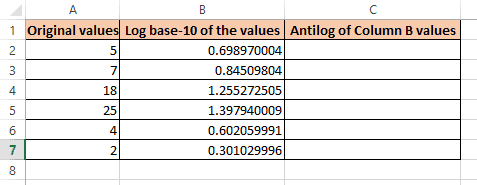Let us see the steps to find the antilogs of the values in column B:

1. Select the first cell where you want the result to be displayed. In our example, select cell C2.
2. Type the formula: =10^B2
3. Press the return key.
4. This will display the antilog of the value B2 in cell C2.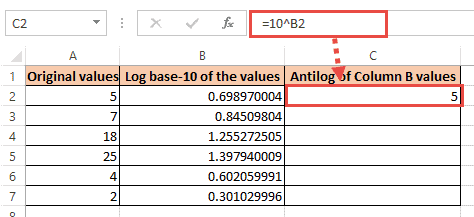5. Copy this formula to the rest of the cells of column C by dragging down the fill handle.

You should now have the antilogs of all values of column B in column C.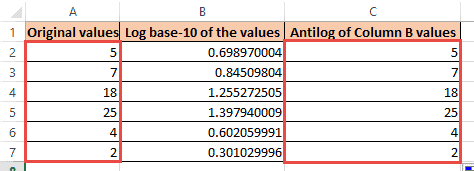Notice from the above image that the results in column C are equivalent to the original values in column A.

This proves that our formula was able to correctly find the inverse of logs of these values.

## How to Calculate Antilog of a Natural Logarithm in Excel

A natural logarithm of a number is its logarithmic value to the base-e.

It is represented using the symbol ‘ln’.

So the natural log of a value, x would be written as ln(x).

It tells us what power we must raise the value of ‘e’, to obtain the number x. Here, ‘e’ is a constant, always having the value 2.7183…

In Excel, we use the LN function to find the natural log of a number.

So if you type: =LN(2.7183) in a given cell, you should get a value close to 1.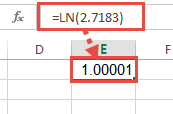Also, if ln(x) = a, then antilog of ‘a’ would be:

`x = e^a`

In Excel, we use the EXP function to find this value.

Let us say you have the following list of values (column A) and their natural logs (column B):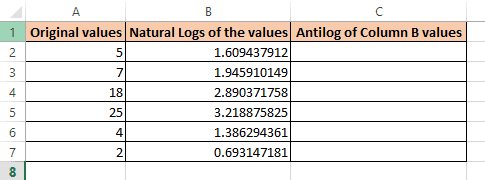Let us see the steps to find the antilogs of the values in column B:

1. Select the first cell where you want the result to be displayed. In our example, select cell C2.
2. Type the formula: =EXP(B2).
3. Press the return key.
4. This will display the antilog of the value B2 in cell C2.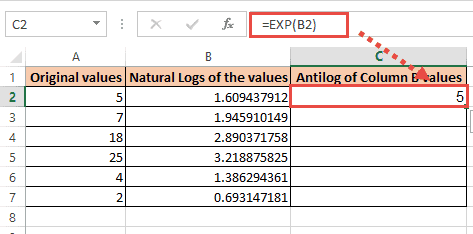5. Copy this formula to the rest of the cells of column C by dragging down the fill handle.

You should now have the antilogs of all values of column B in column C.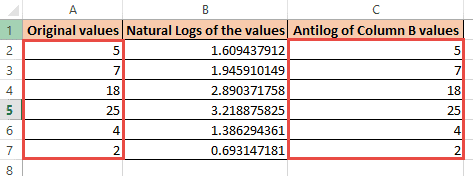Notice from the above image that the results in column C are equivalent to the original values in column A. This proves that our formula was able to correctly find the inverse of natural logs of these values.

In this tutorial, I covered how to find the antilog in Excel.

It covered simple examples with easy explanations, to help you understand and apply the concepts quickly.

I hope you found our explanations easy to understand and our steps easy to follow.

You may also like the following Excel tutorials: